## Single Tuned Circuit:

Consider the Single Tuned Circuit in Fig. 10.21. A tank circuit (i.e. a parallel resonant circuit) on the secondary side is inductively coupled to coil (1) which is excited by a source, υi. Let Rs be the source resistance and R1,R2 be the resistances of coils, 1 and 2, respectively. Also let L1,L2 be the self inductances of the coils, 1 and 2, respectively.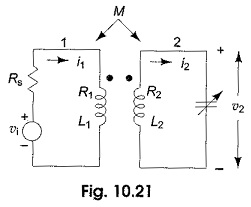Let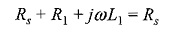with the assumption that Rs >> R1 >> jωL1. The mesh equations for the circuit shown Rs in Fig. 10.21 are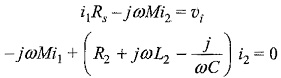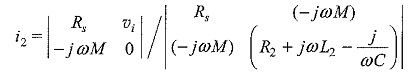or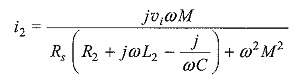The output voltage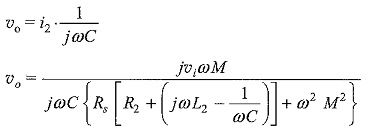The voltage transfer function, or voltage amplification, is given by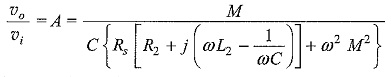When the secondary side is tuned, i.e. when the value of the frequency ω is such that ωL2 = 1/ωC, or at resonance frequency ωr, the amplification is given by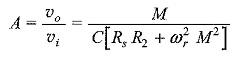the current i2 at resonance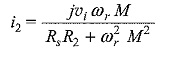Thus, it can be observed that the output voltage, current and amplification depends on the mutual inductance M at resonance frequency, when M = K√L1L2. The maximum output voltage or the maximum amplification depends on M. To get the condition for maximum output voltage, make dυo/dM = 0.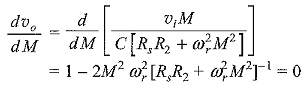From which,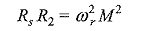or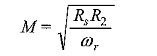From the above value of M, we can calculate the maximum output voltage. Thus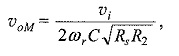or the maximum amplification is given by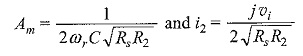The variation of the amplification factor or output voltage with the coefficient of coupling of Single Tuned Circuit is shown in Fig. 10.22.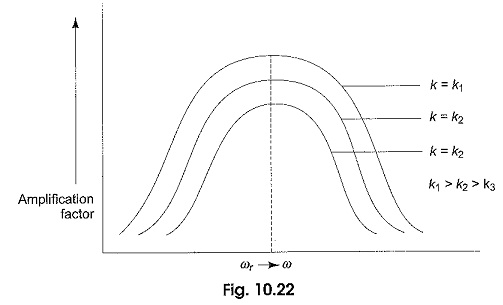Scroll to Top